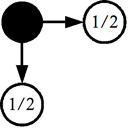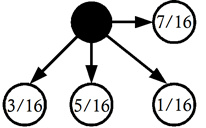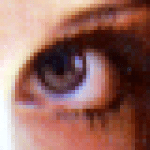# Image Processing Algorithms Part 2: Error Diffusion

This article was originally published in issue 53 of The Crypt Mag

Last time we looked at how to reduce images to 8 colours by finding the nearest colours from the available palette. In part two of this series we will be looking at a technique called error diffusion.

Let’s have a look at our example images again:

And here are the above images reduced to 8 colours from the previous article:

Let’s be honest, the colour reduced versions of the originals are hardly great. To improve things we can employ error diffusion which will cause the image to become dithered and give the illusion of more colours than there really are.

Error diffusion works by comparing the actual colour of a pixel against its nearest colour and taking the difference between them. This difference is known as the error. Portions of the error are split between neighbouring pixels causing the error to be diffused hence the name “error diffusion”.

The simplest form of error diffusion can be shown as:With this form of error diffusion half of the error from the current pixel (represented by the black dot) is diffused to the pixel on the right and half to the pixel below. In a colour image error diffusion should be applied to the red, green and blue channels separately.

An important point to note here is that the total amount of error diffused should never exceed a value of 1. In most cases the total amount of error diffused will equal 1 although I am aware of one type of error diffusion where the total error diffused is less than 1.

It is also important to ensure that when a portion of the error is diffused to neighbouring pixels it does not cause invalid values (e.g. go below 0 or above 255). Should a value go outside of the valid range then it should be truncated (e.g. -10 would be truncated to 0 and 260 would be truncated to 255).

Here is some pseudo-code for the simple error diffusion which should be performed after the current pixel at x,y has been changed to the nearest colour:

```   error = actualColour - nearestColour
PutPixelColour(x+1, y  ) = Truncate(GetPixelColour(x+1, y  ) + 0.5 * error)
PutPixelColour(x  , y+1) = Truncate(GetPixelColour(x  , y+1) + 0.5 * error)
```

The procedure Truncate() in pseudo-code:

```   Procedure Truncate(value)
If value < 0 Then value = 0
If value > 255 Then value = 255
Return value
EndProcedure
```

The quality of this type of error diffusion however is pretty poor and very few people would actually use it. A better one, and arguably the most famous, is Floyd-Steinberg error diffusion. This can be shown as follows:With Floyd-Steinberg error diffusion the error is distributed amongst more neighbouring pixels providing a much nicer overall dithering effect. Here is the Floyd-Steinberg error diffusion in pseudo-code:

```   error = actualColour - nearestColour
PutPixelColour(x+1, y  ) = Truncate(GetPixelColour(x+1, y  ) + 7/16 * error)
PutPixelColour(x-1, y+1) = Truncate(GetPixelColour(x-1, y+1) + 3/16 * error)
PutPixelColour(x  , y+1) = Truncate(GetPixelColour(x  , y+1) + 5/16 * error)
PutPixelColour(x+1, y+1) = Truncate(GetPixelColour(x+1, y+1) + 1/16 * error)
```

Performing the colour reduction with Floyd-Steinberg error diffusion will produce the following results:

As a comparison here are zoomed close-ups of the Lena images:Zoomed close-ups of the original, colour reduced and dithered Lena images

Despite having the same palette I am sure you will agree the colour reduced images with error diffusion look a lot better than the colour reduced images without the error diffusion.

## 8 thoughts on “Image Processing Algorithms Part 2: Error Diffusion”

1.carlos says:

Could you please explain it a bit more? I think I miss something.

I think that you first made a color reduction like in tutorial 1, and then made the error diffusion but In the second step you did not call to FindNearestColour to ensure only 8 unique colors.

Are you using new colors?

1.Francis Loch says:

Hi Carlos,

The colour palette used here is the same as in part one, i.e. black, red, green, yellow, blue, magenta, cyan and white.

As you rightly say, the colour reduction is taken care of by the first part of this tutorial with the error diffusion being applied in the next step. You can think of the process as being something like this:

1. Get colour of pixel.
2. Find nearest colour of pixel from available palette.
3. Replace the pixel with the nearest colour.
4. Calculate the error between the original and nearest colours.
5. Diffuse the error to the neighbouring pixels.

I hope that clarifies things for you, but if not then please let me know.

Kind regards,

Francis

2.carlos says:

Yes, I understand the algorithm but seems that when you diffuse the error to the neighbouring pixels you are not respeting the original palette.

PutPixelColour(x+1, y ) = Truncate(GetPixelColour(x+1, y ) + 7/16 * error)

Is out of the palette.

1.Francis Loch says:

Hi Carlos,

I think I understand what you mean, hopefully this will explain things better.

When you diffuse the error to the neighbouring pixels it can alter the original colours which is necessary for the error diffusion to work. Using the word ‘original’ was a bad choice in my previous explanation to you, so I apologise for that.

Let’s use the simple error diffusion (i.e. half the error to x+1 and half to y+1) as an example, and let’s assume a preselected palette of 0 and 255. If we have a value at (x, y) which is 195, this will be replaced by the nearest colour value which will be 255. Calculating the error between the two values will give us 195-255 = -60.

Now let’s say that the value at (x+1, y) is 130, adding half the error value would give us 130+0.5*(-60) = 100. The same would then be done with the value at (x, y+1).

As you can see the value at (x+1, y) went from being 130 to 100. If the value remained at the original 130 when it is its turn to go through the nearest colour selection it would have become 255. Instead the nearest colour is 0 since the value became 100 with the error diffusion applied.

Kind regards,

Francis

3.caragones says:

Ok, I understand.

Thanks!
.

1.Francis Loch says:

No problem! (^_^)

Kind regards,

Francis

4.Powerpes says:

1.Francis Loch says: